# On joint distributions of order statistics for a discrete case

## Abstract

In this study, the joint distributions of order statistics of innid discrete random variables are expressed. Also, the joint distributions are obtained in the form of an integral. Then, the results related to pf and df are given.

MSC:62G30, 62E15.

## 1 Introduction

The joint probability density function (pdf) and marginal pdf of order statistics of independent but not necessarily identically distributed (innid) random variables was derived by Vaughan and Venables  by means of permanents. In addition, Balakrishnan  and Bapat and Beg  obtained the joint pdf and distribution function (df) of order statistics of innid random variables by means of permanents. Balasubramanian et al.  obtained the distribution of single order statistics in terms of distribution functions of the minimum and maximum order statistics of some subsets of $\left\{{X}_{1},{X}_{2},\dots ,{X}_{n}\right\}$ where ${X}_{i}$’s are innid random variables. Later, Balasubramanian et al.  generalized their previous results  to the case of the joint distribution function of several order statistics. Recurrence relationships among the distribution functions of order statistics arising from innid random variables were obtained by Cao and West . Using multinomial arguments, the pdf of ${X}_{r:n+1}$ ($1\le r\le n+1$) was obtained by Childs and Balakrishnan  by adding another independent random variable to the original n variables ${X}_{1},{X}_{2},\dots ,{X}_{n}$. Also, Balasubramanian et al.  established the identities satisfied by the distributions of order statistics from non-independent non-identical variables through operator methods based on the difference and differential operators. In 1991, Beg  obtained several recurrence relations and identities for product moments of order statistics of innid random variables using permanents. Recently, Cramer et al.  derived the expressions for the distribution and density functions by Ryser’s method and the distributions of maxima and minima based on permanents.

The notion of distribution theory has been applied in various branches of science for investigations. Recently, the notion of a uniform distribution has been applied in sequence spaces and the notion of statistical convergent sequences has been studied and investigated from different aspects by Rath and Tripathy , Tripathy [12, 13], Tripathy and Baruah , Tripathy and Dutta , Tripathy and Sarma , Tripathy and Sen , and others.

A multivariate generalization of classical order statistics for random samples from a continuous multivariate distribution was defined by Corley . Guilbaud  expressed the probability of the functions of innid random vectors as a linear combination of probabilities of the functions of independent and identically distributed (iid) random vectors and thus also for order statistics of random variables. Expressions for generalized joint densities of order statistics of iid random variables in terms of Radon-Nikodym derivatives with respect to product measures based on df were derived by Goldie and Maller .

Several identities and recurrence relations for pdf and df of order statistics of iid random variables were established by numerous authors including Arnold et al. , Balasubramanian and Beg , David , and Reiss . Furthermore, Arnold et al. , David , Gan and Bain , and Khatri  obtained the probability function (pf) and df of order statistics of iid random variables from a discrete parent. Balakrishnan  showed that several relations and identities that have been derived for order statistics from continuous distributions also hold for the discrete case. In 1986, Nagaraja  explored the behavior of higher order conditional probabilities of order statistics in an attempt to understand the structure of discrete order statistics. Later, Nagaraja  considered some results on order statistics of a random sample taken from a discrete population.

In general, the distribution theory for order statistics is complex when the parent distribution is discrete. In this study, the joint distributions of order statistics of innid discrete random variables are expressed in the form of an integral. As far as we know, these approaches have not been considered in the framework of order statistics from innid discrete random variables.

From now on, the subscripts and superscripts are defined at first usage, and these definitions will be valid unless they are redefined.

If ${\mathrm{a}}_{1},{\mathrm{a}}_{2},\dots$ are column vectors, then [$\underset{{m}_{1}}{{\mathrm{a}}_{1}}\phantom{\rule{0.25em}{0ex}}\underset{{m}_{2}}{{\mathrm{a}}_{2}}\phantom{\rule{0.25em}{0ex}}\cdots$] will denote the matrix obtained by taking ${m}_{1}$ copies of ${\mathrm{a}}_{1}$, ${m}_{2}$ copies of ${\mathrm{a}}_{2}$ and so on. perA denotes the permanent of a square matrix A; the permanent is defined just like the determinant, except that all signs in the expansion are positive.

Let ${X}_{1},{X}_{2},\dots ,{X}_{n}$ be innid discrete random variables and ${X}_{1:n}\le {X}_{2:n}\le \cdots \le {X}_{n:n}$ be the order statistics obtained by arranging the n ${X}_{i}$’s in the increasing order of magnitude. Let ${F}_{i}$ and ${f}_{i}$ be df and pf of ${X}_{i}$ ($i=1,2,\dots ,n$), respectively.

The df and pf of ${X}_{{r}_{1}:n},{X}_{{r}_{2}:n},\dots ,{X}_{{r}_{p}:n}$, $1\le {r}_{1}<{r}_{2}<\cdots <{r}_{n}\le n$ ($p=1,2,\dots ,n$) will be given. For notational convenience, we write ${\sum }_{{z}_{1},{z}_{2},\dots ,{z}_{p}}$, ${\sum }_{{m}_{p},{k}_{p},\dots ,{m}_{1},{k}_{1}}$, ∫ and ${\int }_{\mathrm{V}}$ instead of ${\sum }_{{z}_{1}=0}^{{x}_{1}}{\sum }_{{z}_{2}={z}_{1}}^{{x}_{2}}{\sum }_{{z}_{3}={z}_{2}}^{{x}_{3}}\cdots {\sum }_{{z}_{p}={z}_{p-1}}^{{x}_{p}}$, ${\sum }_{{m}_{p}=0}^{n-{r}_{p}}{\sum }_{{k}_{p}=0}^{{r}_{p}-1-{r}_{p-1}}\cdots {\sum }_{{m}_{2}=0}^{{r}_{3}-1-{r}_{2}}{\sum }_{{k}_{2}=0}^{{r}_{2}-1-{r}_{1}}{\sum }_{{m}_{1}=0}^{{r}_{2}-1-{r}_{1}}{\sum }_{{k}_{1}=0}^{{r}_{1}-1}$, ${\int }_{{F}_{{\varsigma }_{2}^{\left(1\right)}}\left({x}_{1}-\right)}^{{F}_{{\varsigma }_{2}^{\left(1\right)}}\left({x}_{1}\right)}{\int }_{{F}_{{\varsigma }_{4}^{\left(1\right)}}\left({x}_{2}-\right)}^{{F}_{{\varsigma }_{4}^{\left(1\right)}}\left({x}_{2}\right)}\cdots {\int }_{{F}_{{\varsigma }_{2p}^{\left(1\right)}}\left({x}_{p}-\right)}^{{F}_{{\varsigma }_{2p}^{\left(1\right)}}\left({x}_{p}\right)}$ and ${\int }_{0}^{{F}_{{\varsigma }_{2}^{\left(1\right)}}\left({x}_{1}\right)}{\int }_{{v}_{{\varsigma }_{2}^{\left(1\right)}}}^{{F}_{{\varsigma }_{4}^{\left(1\right)}}\left({x}_{2}\right)}\cdots {\int }_{{v}_{{\varsigma }_{2\left(p-1\right)}^{\left(1\right)}}}^{{F}_{{\varsigma }_{2p}^{\left(1\right)}}\left({x}_{p}\right)}$ in the expressions below, respectively (${x}_{i}=0,1,2,\dots$) (${z}_{0}=0$).

## 2 Theorems for distribution and probability functions

In this section, the theorems related to pf and df of ${X}_{{r}_{1}:n},{X}_{{r}_{2}:n},\dots ,{X}_{{r}_{p}:n}$ will be given. We will now express the following theorem for the joint pf of order statistics of innid discrete random variables.

Theorem 1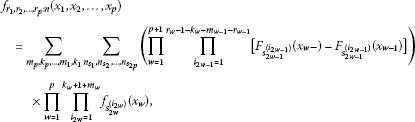(1)

where ${x}_{1}<{x}_{2}<\cdots <{x}_{p}$, ${\sum }_{{n}_{{s}_{1}},{n}_{{s}_{2}},\dots ,{n}_{{s}_{2p}}}$ denotes the sum over ${\bigcup }_{\ell =1}^{2p}{s}_{\ell }$ for which ${s}_{\upsilon }\cap {s}_{\vartheta }=\varphi$ for $\upsilon \ne \vartheta$, $S={\bigcup }_{\ell =1}^{2p+1}{s}_{\ell }$, $S=\left\{1,2,\dots ,n\right\}$, ${r}_{0}=0$, ${r}_{p+1}=n+1$, ${m}_{0}=0$, ${k}_{p+1}=0$, ${m}_{w-1}+{k}_{w}\le {r}_{w}-{r}_{w-1}-1$, ${F}_{{s}_{1}^{\left({i}_{1}\right)}}\left({x}_{0}\right)=0$, ${F}_{{s}_{2p+1}^{\left({i}_{2p+1}\right)}}\left({x}_{p+1}-\right)=1$, ${F}_{i}\left({x}_{w}-\right)=P\left({X}_{i}<{x}_{w}\right)$ ($w=1,2,\dots ,p+1$), ${n}_{{s}_{\ell }}$ is the cardinality of ${s}_{\ell }$ and

${s}_{\ell }=\left\{\begin{array}{cc}\left\{{s}_{\ell }^{\left(1\right)},{s}_{\ell }^{\left(2\right)},\dots ,{s}_{\ell }^{\left({k}_{\frac{\ell }{2}}+\mathrm{1}+{m}_{\frac{\ell }{2}}\right)}\right\},\hfill & \mathit{\text{if}}\phantom{\rule{0.5em}{0ex}}\ell \phantom{\rule{0.5em}{0ex}}\mathit{\text{even}},\hfill \\ \left\{{s}_{\ell }^{\left(1\right)},{s}_{\ell }^{\left(2\right)},\dots ,{s}_{\ell }^{\left({r}_{\frac{\ell +1}{2}}-1-{k}_{\frac{\ell +1}{2}}-{m}_{\frac{\ell -1}{2}}-{r}_{\frac{\ell -1}{2}}\right)}\right\},\hfill & \mathit{\text{if}}\phantom{\rule{0.5em}{0ex}}\ell \phantom{\rule{0.5em}{0ex}}\mathit{\text{odd}}.\hfill \end{array}$

Proof Consider the event $\left\{{X}_{{r}_{1}:n}={x}_{1},{X}_{{r}_{2}:n}={x}_{2},\dots ,{X}_{{r}_{p}:n}={x}_{p}\right\}$.

The above event can be realized in mutually exclusive ways as follows: ${r}_{1}-1-{k}_{1}$ observations are less than ${x}_{1}$, ${k}_{w}+1+{m}_{w}$ ($w=1,2,\dots ,p$) observations are equal to ${x}_{w}$, ${r}_{\xi }-1-{k}_{\xi }-{m}_{\xi -1}-{r}_{\xi -1}$ ($\xi =2,3,\dots ,p$) observations are in interval $\left({x}_{\xi -1},{x}_{\xi }\right)$ and $n-{m}_{p}-{r}_{p}$ observations exceed ${x}_{p}$. The probability function of the above event can be written as

${f}_{{r}_{1},{r}_{2},\dots ,{r}_{p}:n}\left({x}_{1},{x}_{2},\dots ,{x}_{p}\right)=P\left\{{X}_{{r}_{1}:n}={x}_{1},{X}_{{r}_{2}:n}={x}_{2},\dots ,{X}_{{r}_{p}:n}={x}_{p}\right\}.$
(2)

Identity (2) can be expressed as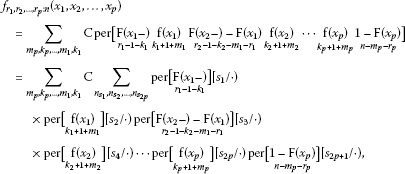where $\mathrm{C}=\left({\prod }_{w=1}^{p+1}{\left[\left({r}_{w}-1-{k}_{w}-{m}_{w-1}-{r}_{w-1}\right)!\right]}^{-1}\right){\prod }_{w=1}^{p}{\left[\left({k}_{w}+1+{m}_{w}\right)!\right]}^{-1}$, $\mathrm{F}\left({x}_{w}\right)={\left({F}_{\mathrm{1}}\left({x}_{w}\right),{F}_{2}\left({x}_{w}\right),\dots ,{F}_{n}\left({x}_{w}\right)\right)}^{\prime }$ and $\mathrm{f}\left({x}_{w}\right)={\left({f}_{1}\left({x}_{w}\right),{f}_{2}\left({x}_{w}\right),\dots ,{f}_{n}\left({x}_{w}\right)\right)}^{\prime }$ are column vectors. $\mathrm{A}\left[{s}_{\ell }/\cdot \right)$ is the matrix obtained from A by taking rows whose indices are in ${s}_{\ell }$. Using the expansion of the permanent in the above identity, we get (1). □

Identity (1) can also be written in the form of an integral as follows.

Theorem 2

${f}_{{r}_{1},{r}_{2},\dots ,{r}_{p}:n}\left({x}_{1},{x}_{2},\dots ,{x}_{p}\right)=\sum _{{n}_{{\varsigma }_{1}},{n}_{{\varsigma }_{2}},\dots ,{n}_{{\varsigma }_{2p}}}\int \left(\prod _{w=1}^{p+1}\prod _{{i}_{w}=1}^{{r}_{w}-{r}_{w-1}-1}\left[{v}_{{\varsigma }_{2w-1}^{\left({i}_{w}\right)}}^{\left(w\right)}-{v}_{{\varsigma }_{2w-1}^{\left({i}_{w}\right)}}^{\left(w-1\right)}\right]\right)\prod _{w=1}^{p}\phantom{\rule{0.2em}{0ex}}d{v}_{{\varsigma }_{2\mathrm{w}}^{\left(1\right)}}^{\left(w\right)},$
(3)

where ${x}_{1}<{x}_{2}<\cdots <{x}_{p}$, ${\sum }_{{n}_{{\varsigma }_{1}},{n}_{{\varsigma }_{2}},\dots ,{n}_{{\varsigma }_{2p}}}$ denotes the sum over ${\bigcup }_{\ell =1}^{2p}{\varsigma }_{\ell }$ for which ${\varsigma }_{\upsilon }\cap {\varsigma }_{\vartheta }=\varphi$ for $\upsilon \ne \vartheta$, $S={\bigcup }_{\ell =1}^{2p+1}{\varsigma }_{\ell }$, ${v}_{{\varsigma }_{2w-1}^{\left({i}_{w}\right)}}^{\left(t\right)}=\left[{v}_{{\varsigma }_{2t}^{\left(1\right)}}^{\left(t\right)}-{F}_{{\varsigma }_{2t}^{\left(1\right)}}\left({x}_{t}-\right)\right]\frac{{f}_{{\varsigma }_{2w-1}^{\left({i}_{w}\right)}}\left({x}_{t}\right)}{{f}_{{\varsigma }_{2t}^{\left(1\right)}}\left({x}_{t}\right)}+{F}_{{\varsigma }_{2w-1}^{\left({i}_{w}\right)}}\left({x}_{t}-\right)$, ${v}_{{\varsigma }_{1}^{\left({i}_{1}\right)}}^{\left(0\right)}=0$, ${v}_{{\varsigma }_{2p+1}^{\left({i}_{p+1}\right)}}^{\left(p+1\right)}=1$ and

${\varsigma }_{\ell }=\left\{\begin{array}{cc}\left\{{\varsigma }_{\ell }^{\left(1\right)}\right\},\hfill & \mathit{\text{if}}\phantom{\rule{0.5em}{0ex}}\ell \phantom{\rule{0.5em}{0ex}}\mathit{\text{even}},\hfill \\ \left\{{\varsigma }_{\ell }^{\left(1\right)},{\varsigma }_{\ell }^{\left(2\right)},\dots ,{\varsigma }_{\ell }^{\left({r}_{\frac{\ell +1}{2}}-{r}_{\frac{\ell -1}{2}}-1\right)}\right\},\hfill & \mathit{\text{if}}\phantom{\rule{0.5em}{0ex}}\ell \phantom{\rule{0.5em}{0ex}}\mathit{\text{odd}}.\hfill \end{array}$

Proof Consider the identity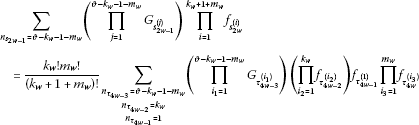(4)

and using (4) in (1), it can be written as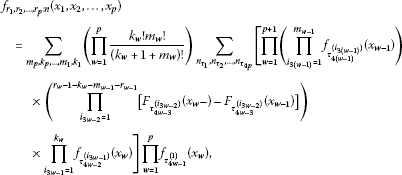where ${\sum }_{{n}_{{\tau }_{1}},{n}_{{\tau }_{2}},\dots ,{n}_{{\tau }_{4p}}}$ denotes the sum over ${\bigcup }_{l=1}^{4p}{\tau }_{l}$ for which ${\tau }_{\upsilon }\cap {\tau }_{\vartheta }=\varphi$ for $\upsilon \ne \vartheta$, $S={\bigcup }_{l=1}^{4p+1}{\tau }_{l}$,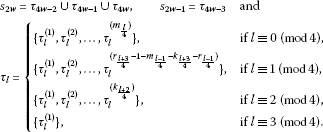The above identity can be written as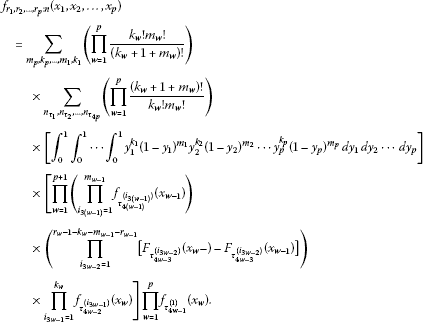The following expression can be written from the above identity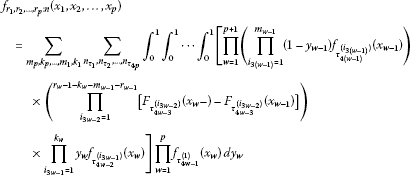and here, if ${v}_{{\tau }_{l}^{\left({i}_{j}\right)}}^{\left(w\right)}={y}_{w}{f}_{{\tau }_{l}^{\left({i}_{j}\right)}}\left({x}_{w}\right)+{F}_{{\tau }_{l}^{\left({i}_{j}\right)}}\left({x}_{w}-\right)$, the following identity is obtained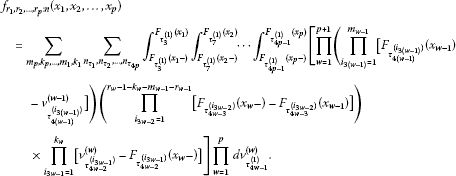(5)

By considering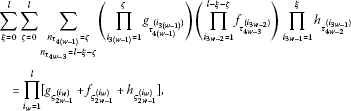(6)

where $\xi +\zeta \le l$, and using (6) for each ${m}_{w-1}$ and ${k}_{w}$ in (5), we getwhere ${\varsigma }_{2w-1}={\tau }_{4\left(w-1\right)}\cup {\tau }_{4w-3}\cup {\tau }_{4w-2}$ and ${\varsigma }_{2w}={\tau }_{4w-1}$. This completes the proof of the theorem. □

We have the following special cases obtained from (3). Consider by taking $p=2$, $n=3$, ${r}_{1}=1$, ${r}_{2}=2$ and ${v}_{{\varsigma }_{5}^{\left(1\right)}}^{\left(2\right)}=\left[{v}_{{\varsigma }_{4}^{\left(1\right)}}^{\left(2\right)}-{F}_{{\varsigma }_{4}^{\left(1\right)}}\left({x}_{2}-\right)\right]\frac{{f}_{{\varsigma }_{5}^{\left(1\right)}}\left({x}_{2}\right)}{{f}_{{\varsigma }_{4}^{\left(1\right)}}\left({x}_{2}\right)}+{F}_{{\varsigma }_{5}^{\left(1\right)}}\left({x}_{2}-\right)$, the following identity is obtained

$\begin{array}{rcl}{f}_{1,2:3}\left({x}_{1},{x}_{2}\right)& =& \sum _{{n}_{{\varsigma }_{2}},{n}_{{\varsigma }_{4}}}{\int }_{{F}_{{\varsigma }_{2}^{\left(1\right)}}\left({x}_{1}-\right)}^{{F}_{{\varsigma }_{2}^{\left(1\right)}}\left({x}_{1}\right)}\left({\int }_{{F}_{{\varsigma }_{4}^{\left(1\right)}}\left({x}_{2}-\right)}^{{F}_{{\varsigma }_{4}^{\left(1\right)}}\left({x}_{2}\right)}\left(1-{v}_{{\varsigma }_{5}^{\left(1\right)}}^{\left(2\right)}\right)\phantom{\rule{0.2em}{0ex}}d{v}_{{\varsigma }_{4}^{\left(1\right)}}^{\left(2\right)}\right)\phantom{\rule{0.2em}{0ex}}d{v}_{{\varsigma }_{2}^{\left(1\right)}}^{\left(1\right)}\\ =& \sum _{{n}_{{\varsigma }_{2}},{n}_{{\varsigma }_{4}}}{f}_{{\varsigma }_{2}^{\left(1\right)}}\left({x}_{1}\right)\left\{{f}_{{\varsigma }_{4}^{\left(1\right)}}\left({x}_{2}\right)+\frac{1}{2}{f}_{{\varsigma }_{5}^{\left(1\right)}}\left({x}_{2}\right){F}_{{\varsigma }_{4}^{\left(1\right)}}\left({x}_{2}-\right)\\ -\frac{1}{2}{f}_{{\varsigma }_{5}^{\left(1\right)}}\left({x}_{2}\right){F}_{{\varsigma }_{4}^{\left(1\right)}}\left({x}_{2}\right)-{f}_{{\varsigma }_{4}^{\left(1\right)}}\left({x}_{2}\right){F}_{{\varsigma }_{5}^{\left(1\right)}}\left({x}_{2}-\right)\right\}\\ =& {f}_{1}\left({x}_{1}\right)\left\{{f}_{2}\left({x}_{2}\right)+\frac{1}{2}{f}_{3}\left({x}_{2}\right){F}_{2}\left({x}_{2}-\right)-\frac{1}{2}{f}_{3}\left({x}_{2}\right){F}_{2}\left({x}_{2}\right)-{f}_{2}\left({x}_{2}\right){F}_{3}\left({x}_{2}-\right)\right\}\\ +{f}_{1}\left({x}_{1}\right)\left\{{f}_{3}\left({x}_{2}\right)+\frac{1}{2}{f}_{2}\left({x}_{2}\right){F}_{3}\left({x}_{2}-\right)-\frac{1}{2}{f}_{2}\left({x}_{2}\right){F}_{3}\left({x}_{2}\right)-{f}_{3}\left({x}_{2}\right){F}_{2}\left({x}_{2}-\right)\right\}\\ +{f}_{2}\left({x}_{1}\right)\left\{{f}_{3}\left({x}_{2}\right)+\frac{1}{2}{f}_{1}\left({x}_{2}\right){F}_{3}\left({x}_{2}-\right)-\frac{1}{2}{f}_{1}\left({x}_{2}\right){F}_{3}\left({x}_{2}\right)-{f}_{3}\left({x}_{2}\right){F}_{1}\left({x}_{2}-\right)\right\}\\ +{f}_{2}\left({x}_{1}\right)\left\{{f}_{1}\left({x}_{2}\right)+\frac{1}{2}{f}_{3}\left({x}_{2}\right){F}_{1}\left({x}_{2}-\right)-\frac{1}{2}{f}_{3}\left({x}_{2}\right){F}_{1}\left({x}_{2}\right)-{f}_{1}\left({x}_{2}\right){F}_{3}\left({x}_{2}-\right)\right\}\\ +{f}_{3}\left({x}_{1}\right)\left\{{f}_{1}\left({x}_{2}\right)+\frac{1}{2}{f}_{2}\left({x}_{2}\right){F}_{1}\left({x}_{2}-\right)-\frac{1}{2}{f}_{2}\left({x}_{2}\right){F}_{1}\left({x}_{2}\right)-{f}_{1}\left({x}_{2}\right){F}_{2}\left({x}_{2}-\right)\right\}\\ +{f}_{3}\left({x}_{1}\right)\left\{{f}_{2}\left({x}_{2}\right)+\frac{1}{2}{f}_{1}\left({x}_{2}\right){F}_{2}\left({x}_{2}-\right)-\frac{1}{2}{f}_{1}\left({x}_{2}\right){F}_{2}\left({x}_{2}\right)-{f}_{2}\left({x}_{2}\right){F}_{1}\left({x}_{2}-\right)\right\}.\end{array}$

Moreover, the above identity in the iid case can be expressed by

${f}_{1,2:3}\left({x}_{1},{x}_{2}\right)=6f\left({x}_{1}\right)f\left({x}_{2}\right)-6f\left({x}_{1}\right)f\left({x}_{2}\right)F\left({x}_{2}\right)+3f\left({x}_{1}\right){f}^{2}\left({x}_{2}\right).$

This result is obtained if $i=1$, $j=2$, and $n=3$ in equation (6) in Khatri .

If ${x}_{1}={x}_{2}=\cdots ={x}_{p}=x$, it should be written as ∫∫∫ instead of ∫ in (3), where ∫∫∫ is to be carried out over the region: ${F}_{{\varsigma }_{2}^{\left(1\right)}}\left({x}_{1}-\right)\le {v}_{{\varsigma }_{2}^{\left(1\right)}}^{\left(1\right)}\le {v}_{{\varsigma }_{4}^{\left(1\right)}}^{\left(2\right)}\le \cdots \le {v}_{{\varsigma }_{2\mathrm{p}}^{\left(1\right)}}^{\left(p\right)}\le {F}_{{\varsigma }_{2\mathrm{p}}^{\left(1\right)}}\left({x}_{p}-\right),{F}_{{\varsigma }_{2}^{\left(1\right)}}\left({x}_{1}-\right)\le {v}_{{\varsigma }_{2}^{\left(1\right)}}^{\left(1\right)}\le {F}_{{\varsigma }_{2}^{\left(1\right)}}\left({x}_{1}\right),{F}_{{\varsigma }_{4}^{\left(1\right)}}\left({x}_{2}-\right)\le {v}_{{\varsigma }_{4}^{\left(1\right)}}^{\left(2\right)}\le {F}_{{\varsigma }_{4}^{\left(1\right)}}\left({x}_{2}\right),\dots ,{F}_{{\varsigma }_{2\mathrm{p}}^{\left(1\right)}}\left({x}_{p}-\right)\le {v}_{{\varsigma }_{2\mathrm{p}}^{\left(1\right)}}^{\left(p\right)}\le {F}_{{\varsigma }_{2\mathrm{p}}^{\left(1\right)}}\left({x}_{p}\right)$.

Further on considering $p=2$, $n=3$, ${r}_{1}=1$, ${r}_{2}=2$, and ${v}_{{\varsigma }_{5}^{\left(1\right)}}^{\left(2\right)}=\left[{v}_{{\varsigma }_{4}^{\left(1\right)}}^{\left(2\right)}-{F}_{{\varsigma }_{4}^{\left(1\right)}}\left({x}_{2}-\right)\right]\frac{{f}_{{\varsigma }_{5}^{\left(1\right)}}\left({x}_{2}\right)}{{f}_{{\varsigma }_{4}^{\left(1\right)}}\left({x}_{2}\right)}+{F}_{{\varsigma }_{5}^{\left(1\right)}}\left({x}_{2}-\right)$ in (3), if ${X}_{1}$, ${X}_{2}$, ${X}_{3}$ are innid discrete random variables and for ${x}_{1}={x}_{2}=x$, then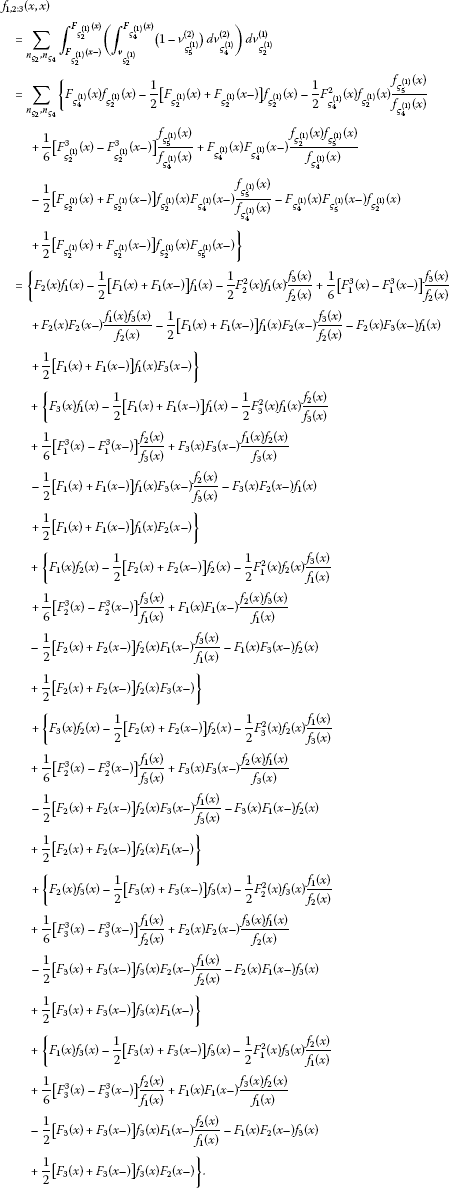Moreover, the above identity in the iid case can be expressed by

$\begin{array}{rc}=& 6F\left(x\right)f\left(x\right)-3\left[F\left(x\right)+F\left(x-\right)\right]f\left(x\right)-3{F}^{2}\left(x\right)f\left(x\right)+\left[{F}^{3}\left(x\right)-{F}^{3}\left(x-\right)\right]+6F\left(x\right)F\left(x-\right)f\left(x\right)\\ -3\left[F\left(x\right)+F\left(x-\right)\right]F\left(x-\right)f\left(x\right)-6F\left(x\right)F\left(x-\right)f\left(x\right)+3\left[F\left(x\right)+F\left(x-\right)\right]f\left(x\right)F\left(x-\right)\\ =& 6F\left(x\right)f\left(x\right)-3F\left(x\right)f\left(x\right)-3F\left(x-\right)f\left(x\right)-3{F}^{2}\left(x\right)f\left(x\right)+{F}^{3}\left(x\right)-{F}^{3}\left(x-\right)\\ =& 3{f}^{2}\left(x\right)-3{F}^{2}\left(x\right)f\left(x\right)+f\left(x\right)\left[3{F}^{2}\left(x\right)-3F\left(x\right)f\left(x\right)+{f}^{2}\left(x\right)\right]\\ =& {f}^{3}\left(x\right)+3{f}^{2}\left(x\right)\left[1-F\left(x\right)\right].\end{array}$

This result is obtained if $r=1$, $s=2$, and $n=3$ in equation (2.4.3) in David .

Furthermore, if ${x}_{1}\le {x}_{2}\le \cdots \le {x}_{p}$, it should be written ∫∫∫ instead of ∫ in (3), where ∫∫∫ is to be carried out over the region: ${v}_{{\varsigma }_{2}^{\left(1\right)}}^{\left(1\right)}\le {v}_{{\varsigma }_{4}^{\left(1\right)}}^{\left(2\right)}\le \cdots \le {v}_{{\varsigma }_{2\mathrm{p}}^{\left(1\right)}}^{\left(p\right)},{F}_{{\varsigma }_{2}^{\left(1\right)}}\left({x}_{1}-\right)\le {v}_{{\varsigma }_{2}^{\left(1\right)}}^{\left(1\right)}\le {F}_{{\varsigma }_{2}^{\left(1\right)}}\left({x}_{1}\right),{F}_{{\varsigma }_{4}^{\left(1\right)}}\left({x}_{2}-\right)\le {v}_{{\varsigma }_{4}^{\left(1\right)}}^{\left(2\right)}\le {F}_{{\varsigma }_{4}^{\left(1\right)}}\left({x}_{2}\right),\dots ,{F}_{{\varsigma }_{2\mathrm{p}}^{\left(1\right)}}\left({x}_{p}-\right)\le {v}_{{\varsigma }_{2\mathrm{p}}^{\left(1\right)}}^{\left(p\right)}\le {F}_{{\varsigma }_{2\mathrm{p}}^{\left(1\right)}}\left({x}_{p}\right)$.

We now express the following theorem to obtain the joint df of order statistics of innid discrete random variables.

Theorem 3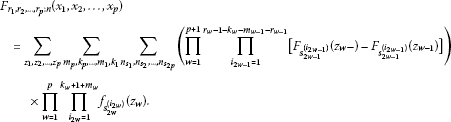(7)

Proof We have

${F}_{{r}_{1},{r}_{2},\dots ,{r}_{p}:n}\left({x}_{1},{x}_{2},\dots ,{x}_{p}\right)=\sum _{{z}_{1},{z}_{2},\dots ,{z}_{p}}{f}_{{r}_{1},{r}_{2},\dots ,{r}_{p}:n}\left({z}_{1},{z}_{2},\dots ,{z}_{p}\right)$
(8)

and using (1) in (8), (7) is obtained. □

The identity (7) can also be written in the form of an integral as follows.

Theorem 4

${F}_{{r}_{1},{r}_{2},\dots ,{r}_{p}:n}\left({x}_{1},{x}_{2},\dots ,{x}_{p}\right)=\sum _{{n}_{{\varsigma }_{1}},{n}_{{\varsigma }_{2}},\dots ,{n}_{{\varsigma }_{2p}}}\left({\int }_{\mathrm{V}}\prod _{w=1}^{p+1}\prod _{{i}_{w}=1}^{{r}_{w}-{r}_{w-1}-1}\left[{v}_{{\varsigma }_{2w-1}^{\left({i}_{w}\right)}}^{\left(w\right)}-{v}_{{\varsigma }_{2w-1}^{\left({i}_{w}\right)}}^{\left(w-1\right)}\right]\right)\prod _{w=1}^{p}\phantom{\rule{0.2em}{0ex}}d{v}_{{\varsigma }_{2\mathrm{w}}^{\left(1\right)}}^{\left(w\right)}.$
(9)

Proof Using (3) in (8), (9) is obtained. □

## 3 Results for distribution and probability functions

In this section, the results related to pf and df of ${X}_{{r}_{1}:n},{X}_{{r}_{2}:n},\dots ,{X}_{{r}_{p}:n}$ will be determined. We express the following result for pf of the r th order statistic of innid discrete random variables.

Result 1

$\begin{array}{rcl}{f}_{{r}_{1}:n}\left({x}_{1}\right)& =& \sum _{{m}_{1}=0}^{n-{r}_{1}}\sum _{{k}_{1}=0}^{{r}_{1}-1}\sum _{{n}_{{s}_{1}},{n}_{{s}_{2}}}\left(\prod _{{i}_{1}=1}^{{r}_{1}-1-{k}_{1}}{F}_{{s}_{1}^{\left({i}_{1}\right)}}\left({x}_{1}-\right)\right)\left(\prod _{{i}_{2}=1}^{{k}_{1}+1+{m}_{1}}{f}_{{s}_{2}^{\left({i}_{2}\right)}}\left({x}_{1}\right)\right)\prod _{{i}_{3}=1}^{n-{m}_{1}-{r}_{1}}\left[1-{F}_{{s}_{3}^{\left({i}_{3}\right)}}\left({x}_{1}\right)\right]\\ =& \sum _{{n}_{{\varsigma }_{1},}{n}_{{\varsigma }_{2}}}{\int }_{{F}_{{\varsigma }_{2}^{\left(1\right)}}\left({x}_{1}-\right)}^{{F}_{{\varsigma }_{2}^{\left(1\right)}}\left({x}_{1}\right)}\left(\prod _{{i}_{1}=1}^{{r}_{1}-1}{v}_{{\varsigma }_{1}^{\left({i}_{1}\right)}}^{\left(1\right)}\right)\left(\prod _{{i}_{2}=1}^{n-{r}_{1}}\left[1-{v}_{{\varsigma }_{3}^{\left({i}_{2}\right)}}^{\left(1\right)}\right]\right)\phantom{\rule{0.2em}{0ex}}d{v}_{{\varsigma }_{2}^{\left(1\right)}}^{\left(1\right)}.\end{array}$
(10)

Proof In (1) and (3), if $p=1$, (10) is obtained. □

In Result 2 and Result 3, the pf’s of minimum and maximum order statistics of innid discrete random variables are given respectively.

Result 2

$\begin{array}{rcl}{f}_{1:n}\left({x}_{1}\right)& =& \sum _{{m}_{1}=0}^{n-1}\sum _{{n}_{{s}_{2}}}\left(\prod _{{i}_{2}=1}^{1+{m}_{1}}{f}_{{s}_{2}^{\left({i}_{2}\right)}}\left({x}_{1}\right)\right)\prod _{{i}_{3}=1}^{n-{m}_{1}-1}\left[1-{F}_{{s}_{3}^{\left({i}_{3}\right)}}\left({x}_{1}\right)\right]\\ =& \sum _{{n}_{{\varsigma }_{2}}}{\int }_{{F}_{{\varsigma }_{2}^{\left(1\right)}}\left({x}_{1}-\right)}^{{F}_{{\varsigma }_{2}^{\left(1\right)}}\left({x}_{1}\right)}\left(\prod _{{i}_{2}=1}^{n-1}\left[1-{v}_{{\varsigma }_{3}^{\left({i}_{2}\right)}}^{\left(1\right)}\right]\right)\phantom{\rule{0.2em}{0ex}}d{v}_{{\varsigma }_{2}^{\left(1\right)}}^{\left(1\right)}.\end{array}$
(11)

Proof In (10), if ${r}_{1}=1$, (11) is obtained. □

Result 3

$\begin{array}{rcl}{f}_{n:n}\left({x}_{1}\right)& =& \sum _{{k}_{1}=0}^{n-1}\sum _{{n}_{{s}_{1}},{n}_{{s}_{2}}}\left(\prod _{{i}_{1}=1}^{n-1-{k}_{1}}{F}_{{s}_{1}^{\left({i}_{1}\right)}}\left({x}_{1}-\right)\right)\prod _{{i}_{2}=1}^{{k}_{1}+1}{f}_{{s}_{2}^{\left({i}_{2}\right)}}\left({x}_{1}\right)\\ =& \sum _{{n}_{{\varsigma }_{1}},{n}_{{\varsigma }_{2}}}{\int }_{{F}_{{\varsigma }_{2}^{\left(1\right)}}\left({x}_{1}-\right)}^{{F}_{{\varsigma }_{2}^{\left(1\right)}}\left({x}_{1}\right)}\left(\prod _{{i}_{1}=1}^{n-1}{v}_{{\varsigma }_{1}^{\left({i}_{1}\right)}}^{\left(1\right)}\right)\phantom{\rule{0.2em}{0ex}}d{v}_{{\varsigma }_{2}^{\left(1\right)}}^{\left(1\right)}.\end{array}$
(12)

Proof In (10), if ${r}_{1}=n$, (12) is obtained. □

In the following result, we determine the joint pf of ${X}_{1:n},{X}_{2:n},\dots ,{X}_{p:n}$.

Result 4 If ${x}_{1}\le {x}_{2}\le \cdots \le {x}_{p}$,

$\begin{array}{rcl}{f}_{1,2,\dots ,p:n}\left({x}_{1},{x}_{2},\dots ,{x}_{p}\right)& =& \sum _{{m}_{p}=0}^{n-p}\sum _{{n}_{{s}_{2}},{n}_{{s}_{4}},\dots ,{n}_{{s}_{2p}}}\left(\prod _{{i}_{2p+1}=1}^{n-{m}_{p}-p}\left[1-{F}_{{s}_{2p+1}^{\left({i}_{2p+1}\right)}}\left({x}_{p}\right)\right]\right)\prod _{w=1}^{p}\prod _{{i}_{2w}=1}^{1+{m}_{w}}{f}_{{s}_{2w}^{\left({i}_{2w}\right)}}\left({x}_{w}\right)\\ =& \sum _{{n}_{{\varsigma }_{2}},{n}_{{\varsigma }_{4}},\dots ,{n}_{{\varsigma }_{2p}}}\int \int \cdots \int \left(\prod _{{i}_{p+1}=1}^{n-p}\left[1-{v}_{{\varsigma }_{2p+1}^{\left({i}_{p+1}\right)}}^{\left(p\right)}\right]\right)\prod _{w=1}^{p}\phantom{\rule{0.2em}{0ex}}d{v}_{{\varsigma }_{2\mathrm{w}}^{\left(1\right)}}^{\left(w\right)},\end{array}$
(13)

where ∫∫is to be carried out over the region: ${v}_{{\varsigma }_{2}^{\left(1\right)}}^{\left(1\right)}\le {v}_{{\varsigma }_{4}^{\left(1\right)}}^{\left(2\right)}\le \cdots \le {v}_{{\varsigma }_{2\mathrm{p}}^{\left(1\right)}}^{\left(p\right)},{F}_{{\varsigma }_{2}^{\left(1\right)}}\left({x}_{1}-\right)\le {v}_{{\varsigma }_{2}^{\left(1\right)}}^{\left(1\right)}\le {F}_{{\varsigma }_{2}^{\left(1\right)}}\left({x}_{1}\right),{F}_{{\varsigma }_{4}^{\left(1\right)}}\left({x}_{2}-\right)\le {v}_{{\varsigma }_{4}^{\left(1\right)}}^{\left(2\right)}\le {F}_{{\varsigma }_{4}^{\left(1\right)}}\left({x}_{2}\right),\dots ,{F}_{{\varsigma }_{2\mathrm{p}}^{\left(1\right)}}\left({x}_{p}-\right)\le {v}_{{\varsigma }_{2\mathrm{p}}^{\left(1\right)}}^{\left(p\right)}\le {F}_{{\varsigma }_{2\mathrm{p}}^{\left(1\right)}}\left({x}_{p}\right)$.

Proof In (1) and (3), if ${r}_{1}=1$, ${r}_{2}=2,\dots ,{r}_{p}=p$ and ∫∫∫ instead of ∫, (13) is obtained. □

Specially, in (13), by taking $p=2$, $n=3$ and ${v}_{{\varsigma }_{5}^{\left(1\right)}}^{\left(2\right)}=\left[{v}_{{\varsigma }_{4}^{\left(1\right)}}^{\left(2\right)}-{F}_{{\varsigma }_{4}^{\left(1\right)}}\left({x}_{2}-\right)\right]\frac{{f}_{{\varsigma }_{5}^{\left(1\right)}}\left({x}_{2}\right)}{{f}_{{\varsigma }_{4}^{\left(1\right)}}\left({x}_{2}\right)}+{F}_{{\varsigma }_{5}^{\left(1\right)}}\left({x}_{2}-\right)$, the following identity is obtained

$\begin{array}{rcl}{f}_{1,2:3}\left({x}_{1},{x}_{2}\right)& =& \sum _{{n}_{{s}_{2}},{n}_{{s}_{4}}}\left(1-{F}_{{s}_{5}^{\left(1\right)}}\left({x}_{2}\right)\right){f}_{{s}_{2}^{\left(1\right)}}\left({x}_{1}\right){f}_{{s}_{4}^{\left(1\right)}}\left({x}_{2}\right)+\sum _{{n}_{{s}_{2}}}{f}_{{s}_{2}^{\left(1\right)}}\left({x}_{1}\right){f}_{{s}_{4}^{\left(1\right)}}\left({x}_{2}\right){f}_{{s}_{4}^{\left(2\right)}}\left({x}_{2}\right)\\ =& \left(1-{F}_{1}\left({x}_{2}\right)\right){f}_{2}\left({x}_{1}\right){f}_{3}\left({x}_{2}\right)+\left(1-{F}_{1}\left({x}_{2}\right)\right){f}_{3}\left({x}_{1}\right){f}_{2}\left({x}_{2}\right)+\left(1-{F}_{2}\left({x}_{2}\right)\right){f}_{3}\left({x}_{1}\right){f}_{1}\left({x}_{2}\right)\\ +\left(1-{F}_{2}\left({x}_{2}\right)\right){f}_{1}\left({x}_{1}\right){f}_{3}\left({x}_{2}\right)+\left(1-{F}_{3}\left({x}_{2}\right)\right){f}_{1}\left({x}_{1}\right){f}_{2}\left({x}_{2}\right)\\ +\left(1-{F}_{3}\left({x}_{2}\right)\right){f}_{2}\left({x}_{1}\right){f}_{1}\left({x}_{2}\right)+{f}_{1}\left({x}_{2}\right){f}_{2}\left({x}_{2}\right){f}_{3}\left({x}_{1}\right)\\ +{f}_{2}\left({x}_{2}\right){f}_{1}\left({x}_{2}\right){f}_{3}\left({x}_{1}\right)+{f}_{3}\left({x}_{2}\right){f}_{1}\left({x}_{2}\right){f}_{2}\left({x}_{1}\right).\end{array}$

Moreover, the above identity in the iid case can be expressed as

${f}_{1,2:3}\left({x}_{1},{x}_{2}\right)=6f\left({x}_{1}\right)f\left({x}_{2}\right)-6f\left({x}_{1}\right)f\left({x}_{2}\right)F\left({x}_{2}\right)+3f\left({x}_{1}\right){f}^{2}\left({x}_{2}\right).$

We now establish three results for the df of single order statistic of innid discrete random variables.

Result 5

$\begin{array}{rcl}{F}_{{r}_{1}:n}\left({x}_{1}\right)& =& \sum _{{z}_{1}=0}^{{x}_{1}}\sum _{{m}_{1}=0}^{n-{r}_{1}}\sum _{{k}_{1}=0}^{{r}_{1}-1}\sum _{{n}_{{s}_{1}},{n}_{{s}_{2}}}\left(\prod _{{i}_{1}=1}^{{r}_{1}-1-{k}_{1}}{F}_{{s}_{1}^{\left({i}_{1}\right)}}\left({z}_{1}-\right)\right)\left(\prod _{{i}_{2}=1}^{{k}_{1}+1+{m}_{1}}{f}_{{s}_{2}^{\left({i}_{2}\right)}}\left({z}_{1}\right)\right)\prod _{{i}_{3}=1}^{n-{m}_{1}-{r}_{1}}\left[1-{F}_{{s}_{3}^{\left({i}_{3}\right)}}\left({z}_{1}\right)\right]\\ =& \sum _{{n}_{{\varsigma }_{1},}{n}_{{\varsigma }_{2}}}{\int }_{0}^{{F}_{{\varsigma }_{2}^{\left(1\right)}}\left({x}_{1}\right)}\left(\prod _{{i}_{1}=1}^{{r}_{1}-1}{v}_{{\varsigma }_{1}^{\left({i}_{1}\right)}}^{\left(1\right)}\right)\left(\prod _{{i}_{2}=1}^{n-{r}_{1}}\left[1-{v}_{{\varsigma }_{3}^{\left({i}_{2}\right)}}^{\left(1\right)}\right]\right)\phantom{\rule{0.2em}{0ex}}d{v}_{{\varsigma }_{2}^{\left(1\right)}}^{\left(1\right)}.\end{array}$
(14)

Proof In (7) and (9), if $p=1$, (14) is obtained. □

Result 6

$\begin{array}{rcl}{F}_{1:n}\left({x}_{1}\right)& =& \sum _{{z}_{1}=0}^{{x}_{1}}\sum _{{m}_{1}=0}^{n-1}\sum _{{n}_{{s}_{2}}}\left(\prod _{{i}_{2}=1}^{1+{m}_{1}}{f}_{{s}_{2}^{\left({i}_{2}\right)}}\left({z}_{1}\right)\right)\prod _{{i}_{3}=1}^{n-{m}_{1}-1}\left[1-{F}_{{s}_{3}^{\left({i}_{3}\right)}}\left({z}_{1}\right)\right]\\ =& \sum _{{n}_{{\varsigma }_{2}}}{\int }_{0}^{{F}_{{\varsigma }_{2}^{\left(1\right)}}\left({x}_{1}\right)}\left(\prod _{{i}_{2}=1}^{n-1}\left[1-{v}_{{\varsigma }_{3}^{\left({i}_{2}\right)}}^{\left(1\right)}\right]\right)\phantom{\rule{0.2em}{0ex}}d{v}_{{\varsigma }_{2}^{\left(1\right)}}^{\left(1\right)}.\end{array}$
(15)

Proof In (14), if ${r}_{1}=1$, (15) is obtained. □

Result 7

$\begin{array}{rcl}{F}_{n:n}\left({x}_{1}\right)& =& \sum _{{z}_{1}=0}^{{x}_{1}}\sum _{{k}_{1}=0}^{n-1}\sum _{{n}_{{s}_{1}},{n}_{{s}_{2}}}\left(\prod _{{i}_{1}=1}^{n-1-{k}_{1}}{F}_{{s}_{1}^{\left({i}_{1}\right)}}\left({z}_{1}-\right)\right)\prod _{{i}_{2}=1}^{{k}_{1}+1}{f}_{{s}_{2}^{\left({i}_{2}\right)}}\left({z}_{1}\right)\\ =& \sum _{{n}_{{\varsigma }_{1}},{n}_{{\varsigma }_{2}}}{\int }_{0}^{{F}_{{\varsigma }_{2}^{\left(1\right)}}\left({x}_{1}\right)}\left(\prod _{{i}_{1}=1}^{n-1}{v}_{{\varsigma }_{1}^{\left({i}_{1}\right)}}^{\left(1\right)}\right)\phantom{\rule{0.2em}{0ex}}d{v}_{{\varsigma }_{2}^{\left(1\right)}}^{\left(1\right)}.\end{array}$
(16)

Proof In (14), if ${r}_{1}=n$, (16) is obtained. □

## References

1. Vaughan RJ, Venables WN: Permanent expressions for order statistics densities. J. R. Stat. Soc. B 1972, 34: 308–310.

2. Balakrishnan N: Permanents order statistics, outliers and robustness. Rev. Mat. Complut. 2007, 20: 7–107.

3. Bapat RB, Beg MI: Order statistics for nonidentically distributed variables and permanents. Sankhya, Ser. A 1989, 51: 79–93.

4. Balasubramanian K, Beg MI, Bapat RB: On families of distributions closed under extrema. Sankhya, Ser. A 1991, 53: 375–388.

5. Balasubramanian K, Beg MI, Bapat RB: An identity for the joint distribution of order statistics and its applications. J. Stat. Plan. Inference 1996, 55: 13–21. 10.1016/0378-3758(95)00138-7

6. Cao G, West M: Computing distributions of order statistics. Commun. Stat., Theory Methods 1997, 26: 755–764. 10.1080/03610929708831947

7. Childs A, Balakrishnan N: Relations for order statistics from non-identical logistic random variables and assessment of the effect of multiple outliers on bias of linear estimators. J. Stat. Plan. Inference 2006, 136: 2227–2253. 10.1016/j.jspi.2005.08.026

8. Balasubramanian K, Balakrishnan N, Malik HJ: Identities for order statistics from non-independent non-identical variables. Sankhya, Ser. B 1994, 56: 67–75.

9. Beg MI: Recurrence relations and identities for product moments of order statistics corresponding to nonidentically distributed variables. Sankhya, Ser. A 1991, 53: 365–374.

10. Cramer E, Herle K, Balakrishnan N: Permanent expansions and distributions of order statistics in the INID case. Commun. Stat., Theory Methods 2009, 38: 2078–2088. 10.1080/03610920802311725

11. Rath D, Tripathy BC: Matrix maps on sequence spaces associated with sets of integers. Indian J. Pure Appl. Math. 1996, 27(2):197–206.

12. Tripathy BC: Matrix transformations between some classes of sequences. J. Math. Anal. Appl. 2001, 206: 448–450.

13. Tripathy BC: On generalized difference paranormed statistically convergent sequences. Indian J. Pure Appl. Math. 2004, 35(5):655–663.

14. Tripathy BC, Baruah A: Lacunary statistical convergent and lacunary strongly convergent generalized difference sequences of fuzzy real numbers. Kyungpook Math. J. 2010, 50(4):565–574. 10.5666/KMJ.2010.50.4.565

15. Tripathy BC, Dutta H: On some lacunary difference sequence spaces defined by a sequence of Orlicz functions and q -lacunary ${\mathrm{\Delta }}_{m}^{n}$ -statistical convergence. An. Stiint. Univ. Ovidius, Ser. Mat. 2012, 20(1):417–430.

16. Tripathy BC, Sarma B: Statistically convergent difference double sequence spaces. Acta Math. Sin. 2008, 24(5):737–742. 10.1007/s10114-007-6648-0

17. Tripathy BC, Sen M: On generalized statistically convergent sequences. Indian J. Pure Appl. Math. 2001, 32(11):1689–1964.

18. Corley HW: Multivariate order statistics. Commun. Stat., Theory Methods 1984, 13: 1299–1304. 10.1080/03610928408828756

19. Guilbaud O: Functions of non-i.i.d. random vectors expressed as functions of i.i.d. random vectors. Scand. J. Stat. 1982, 9: 229–233.

20. Goldie CM, Maller RA: Generalized densities of order statistics. Stat. Neerl. 1999, 53: 222–246. 10.1111/1467-9574.00107

21. Arnold BC, Balakrishnan N, Nagaraja HN: A First Course in Order Statistics. Wiley, New York; 1992.

22. Balasubramanian K, Beg MI: On special linear identities for order statistics. Statistics 2003, 37: 335–339. 10.1080/715019246

23. David HA: Order Statistics. Wiley, New York; 1970.

24. Reiss R-D: Approximate Distributions of Order Statistics. Springer, New York; 1989.

25. Gan G, Bain LJ: Distribution of order statistics for discrete parents with applications to censored sampling. J. Stat. Plan. Inference 1995, 44: 37–46. 10.1016/0378-3758(95)92781-5

26. Khatri CG: Distributions of order statistics for discrete case. Ann. Inst. Stat. Math. 1962, 14: 167–171. 10.1007/BF02868637

27. Balakrishnan N: Order statistics from discrete distributions. Commun. Stat., Theory Methods 1986, 15: 657–675. 10.1080/03610928608829144

28. Nagaraja HN: Structure of discrete order statistics. J. Stat. Plan. Inference 1986, 13: 165–177.

29. Nagaraja HN: Order statistics from discrete distributions. Statistics 1992, 23: 189–216. 10.1080/02331889208802365

## Author information

Authors

### Corresponding author

Correspondence to M Güngör.

### Competing interests

The authors declare that they have no competing interests.

### Authors’ contributions

MG, YB and BY have contributed to all parts of the article. All authors read and approved the final manuscript.

## Rights and permissions

Reprints and Permissions

Güngör, M., Bulut, Y. & Yüzbaşı, B. On joint distributions of order statistics for a discrete case. J Inequal Appl 2012, 264 (2012). https://doi.org/10.1186/1029-242X-2012-264

• Accepted:

• Published:

• DOI: https://doi.org/10.1186/1029-242X-2012-264

### Keywords

• order statistics
• discrete random variable
• probability function
• distribution function